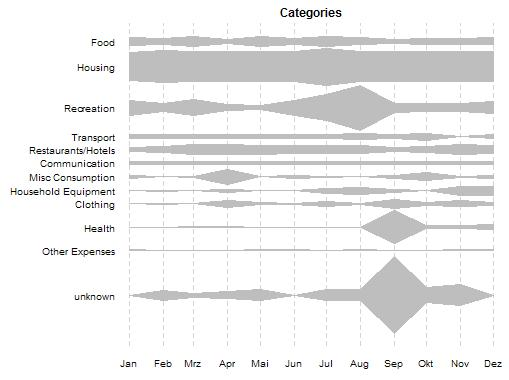## 2012-01-28

### Categorizing my expenses

In order to analyse my expenses, a classification scheme is necessary. I need to identify categories that are meaningful to me. I decided to go with the “Classification of Individual Consumption by Purpose” (COICOP), for three reasons:

• It is made by people who have thought more about consumption classification than I ever will.
• It is feasible to assign bank transactions and tracked cash spendings to one of the 12 top level categories.
• It is widely used by statistics divisions, e.g. the Federal Statistical Office of Germany, Eurostat, and the UN. This means I can do social comparisons: In which categories do I spend more money than the average? Do the prices I pay rise faster than the price indices suggest?

So I classified my last year’s expense data according to COICOP. Here is a chart showing the portions of the categories for each month:For me, the holidays, prepared in August and traveled in September (shown as unknown expenses), are much more dominant than I expected. Except for the new glasses in September I did not make any larger investments.

I like this kind of chart more than stacked bar charts because the history for each category is very visible. This chart is called inkblot chart. I stumbled on it on junk charts, asked how to implement it in R on StackOverflow, and included a revised version in the latest `pft` package. See below for more information.

You find the `inkblot` function in the `pft` package. It is work in progress. The current version is experimental and I did not even try to submit it to CRAN. You can inspect it by downloading it from my personal repository:

``````> install.packages(
+   "pft",
+   repos=c(getOption("repos"),
+      "http://userpage.fu-berlin.de/~kweinert/R"),
+   dependencies=c("Depends", "Suggests")
+ )``````

If you are not a Windows user, you may have to add the `type="source"` argument. Or you can copy the function from below:

``````> inkblot
function(series, names.arg= NULL, col="grey", border=par("fg"), main=NULL,
na.replace=FALSE, min.height=NULL,
major.ticks = "auto", major.format="",
grid=TRUE, lty.grid="dashed", col.grid="lightgrey",
verbose=getOption("verbose"), ...) {
if(!xtsible(series)) stop("invalid 'series' argument, xts compatible expected.")
if(!na.replace)
series <- na.fail(series)
else
series[which(is.na(series))] <- 0

if(any(series < 0)) stop("invalid 'series' argument, non-negative values expected.")
if(is.null(names.arg)) names.arg <- colnames(series)
if(major.format=="") major.format="%Y"  # hack for roxygen
if(length(col)!=dim(series)) col <- rep(col, length.out=dim(series))
old.par <- par(no.readonly = TRUE);on.exit(par(old.par))
par(...)

if(is.null(min.height)) {
ytotal <- 0
for(i in 1:dim(series)) ytotal <- ytotal + max(series[, i])
min.height <- ytotal * par("cex") / (1.5 * dim(series))
}
if(verbose) cat("min.height is ", min.height, "\n")

ytotal <- 0
for(i in 1:dim(series)) {
ytotal <- ytotal + max(series[, i], min.height)
}
if(verbose) cat("ytotal is ", ytotal, "\n")

x <- xy.coords(.index(series), series[, 1])\$x
plot(x, 1:length(x), type="n", ylim=c(0,1)*ytotal, yaxt="n", xaxt="n", bty="n", ylab="", xlab="", main=main)
ep <- axTicksByTime(series, ticks.on=major.ticks, k=1, labels=TRUE, format.labels=major.format)
axis(side=1, at = x[ep], labels = names(ep), las=1, tick=FALSE)
if (grid) abline(v=x[ep], col=col.grid, lty=lty.grid)

catNumber <- 1
offset <- 0
res <- NULL
for(catNumber in 1:dim(series)) {
y <- 0.5 * as.vector(series[,catNumber])
offset <- offset + max(max(y), 0.5*min.height)
polygon(c(x, rev(x)), c(offset+y, offset-rev(y)), col=col[catNumber], border=border)
if(!is.null(names.arg)) mtext(text=names.arg[catNumber], side=2, line=0, at=offset, las=2, adj=1, cex=par("cex")*par("cex.axis"))
res <- c(res, offset)
offset <- offset + max(max(abs(y[!is.na(y)])), 0.5*min.height)
catNumber <- catNumber + 1
}
return(res)
}``````

This post has been submitted to R bloggers, a RSS aggregator for R news and tutorials. If you are interested in R, check it out!